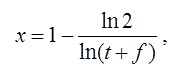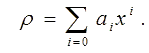### Electron Impact Ionization (EII) Rate Coefficient

The total EII rate coefficient averaged over a Maxwell-Boltzmann electron energy distribution at temperature T is given by the Burgess-Tully type scaling. The temperature T is scaled aswhere t = kbT/E0 for electron energy E0 and f is a constant scaling parameter. The rate coefficient is scaled aswhere E1 the first exponential integral.
The scaled rate coefficient is fitted by the polynomial form